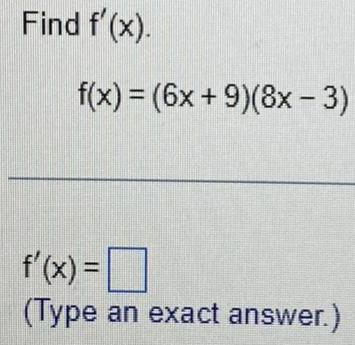Calculus
Differentiation
Find f'(x). f(x) = (6x + 9)(8x - 3) f'(x) = (Type an exact answer.)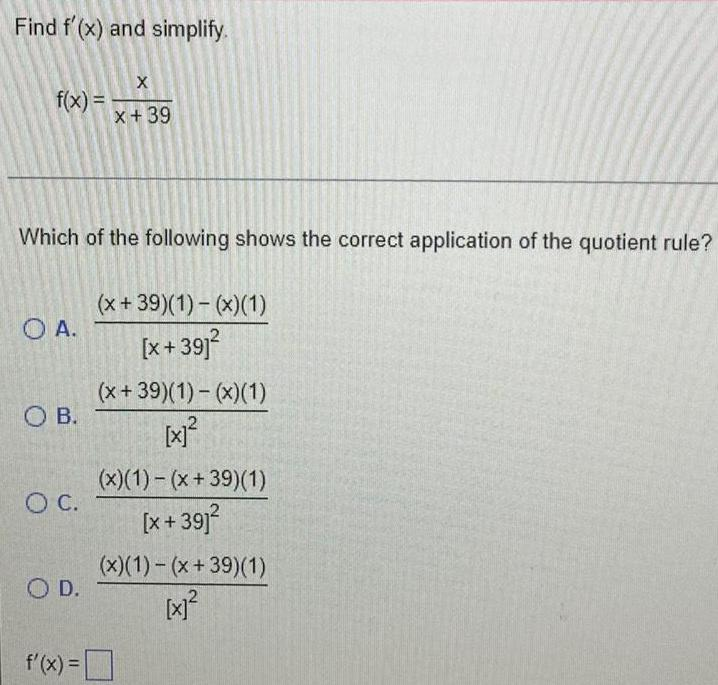Calculus
Differentiation
Find f'(x) and simplify. f(x) = x / x +39 Which of the following shows the correct application of the quotient rule? A.(x +39)(1)-(x)(1)/[x+39]² B. (x +39)(1)-(x)(1)/[x]² C.(x)(1)-(x +39)(1)/[x+39]² D. (x)(1)-(x +39)(1)/[x]² f'(x) =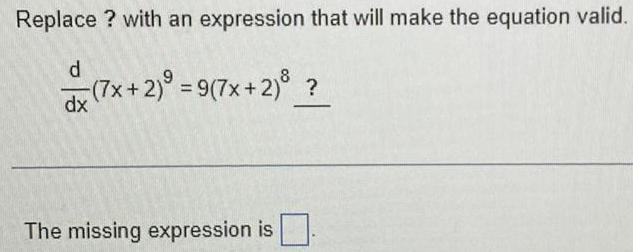Calculus
Differentiation
Replace ? with an expression that will make the equation valid. d/dx (7x+2)⁹ = 9(7x+2)⁸ ? The missing expression is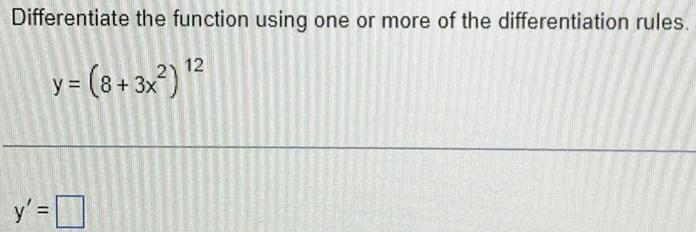Calculus
Differentiation
Differentiate the function using one or more of the differentiation rules. y = (8+3x²)¹² y'=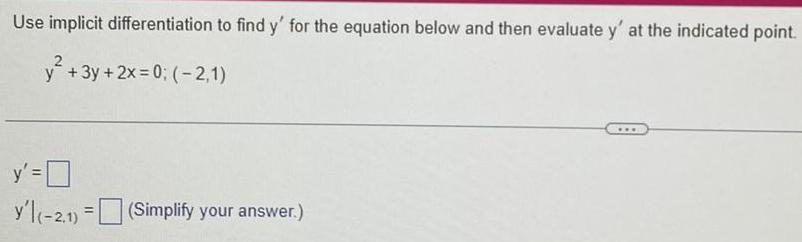Calculus
Differentiation
Use implicit differentiation to find y' for the equation below and then evaluate y' at the indicated point. y²+3y + 2x=0; (-2,1) y' = y'l(-2,1) = (Simplify your answer.)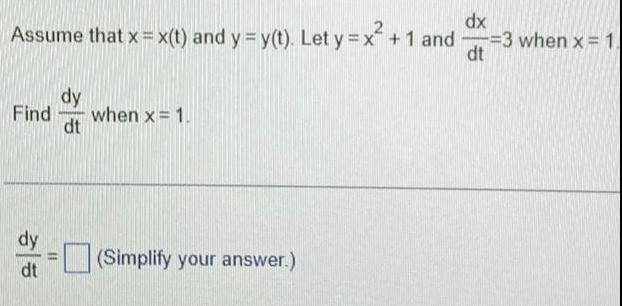Calculus
Differentiation
Assume that x = x(t) and y = y(t). Let y = x² +1 and dx/dt = 3 when x = 1. Find dy/dt when x = 1. dy/dt = (Simplify your answer.)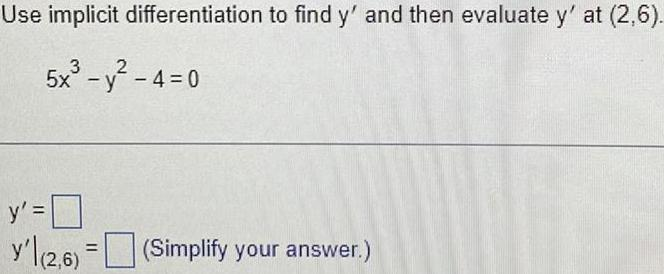Calculus
Differentiation
Use implicit differentiation to find y' and then evaluate y' at (2,6). 5x³-y²-4=0 y' = y'|(2,6) (Simplify your answer.)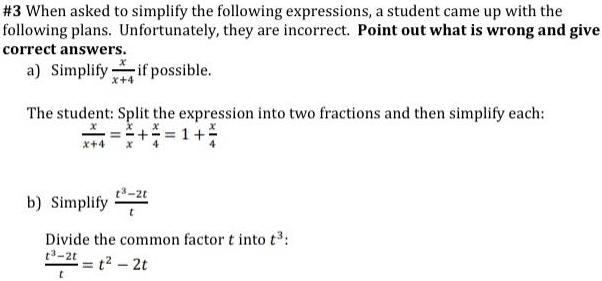Calculus
Differentiation
When asked to simplify the following expressions, a student came up with the following plans. Unfortunately, they are incorrect. Point out what is wrong and give correct answers. a) Simplify x/x+4 if possible. The student: Split the expression into two fractions and then simplify each: x/ x+4 = x/x + x/4 = 1 + x/4 b) Simplify t³-2t/ t Divide the common factor t into t³: t³-2t / t = t² - 2t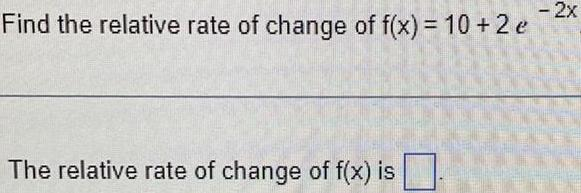Calculus
Differentiation
Find the relative rate of change of f(x) = 10 + 2e^-2x The relative rate of change of f(x) is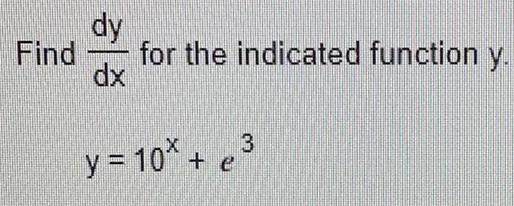Calculus
Differentiation
Find dy/dx for the indicated function y. y = 10x + e³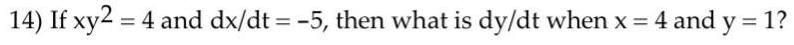Calculus
Differentiation
If xy² = 4 and dx/dt = -5, then what is dy/dt when x = 4 and y = 1?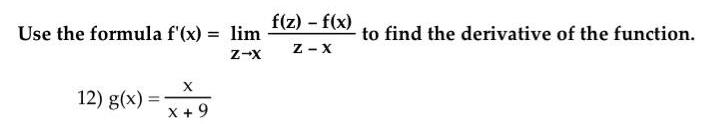Calculus
Differentiation
Use the formula f'(x) = lim z→x f(z) - f(x) / (z-x) to find the derivative of the function. g(x) = x/ (x+ 9)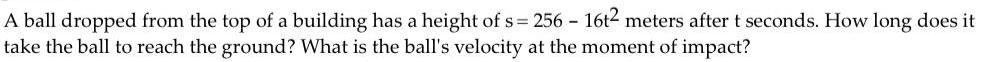Calculus
Differentiation
A ball dropped from the top of a building has a height of s= 256 - 16t² meters after t seconds. How long does it take the ball to reach the ground? What is the ball's velocity at the moment of impact?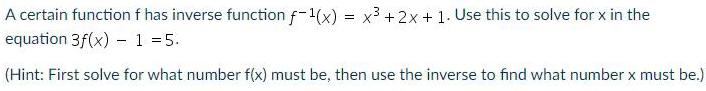Calculus
Differentiation
A certain function f has inverse function f⁻¹(x) = x³ + 2x + 1. Use this to solve for x in the equation 3f(x) - 1 = 5. (Hint: First solve for what number f(x) must be, then use the inverse to find what number x must be.)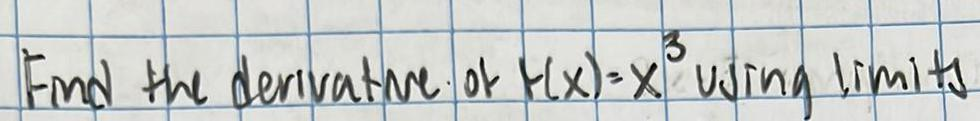Calculus
Differentiation
Find the derivative of f(x)=x³ using limits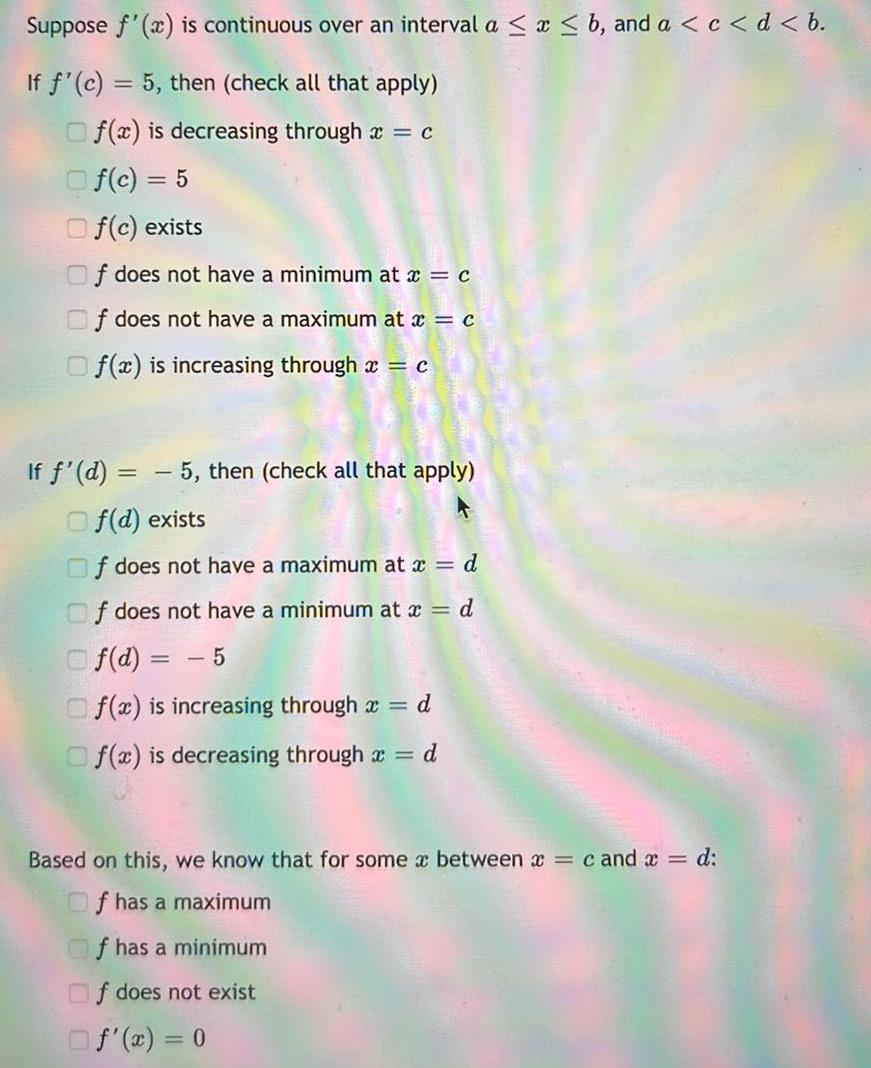Calculus
Differentiation
Suppose f'(x) is continuous over an interval α ≤ x ≤ b, and a < c < d < b. If f'(c) = 5, then (check all that apply) f(x) is decreasing through x = c f(c) = 5 f(c) exists f does not have a minimum at x = c f does not have a maximum at x = c f(x) is increasing through x = c If f'(d) = -5, then (check all that apply) f(d) exists f does not have a maximum at x = d f does not have a minimum at x = d f(d) = -5 f(x) is increasing through x = d f(x) is decreasing through x = d Based on this, we know that for some x between x = c and x = d: f has a maximum f has a minimum f does not exist f'(x) = 0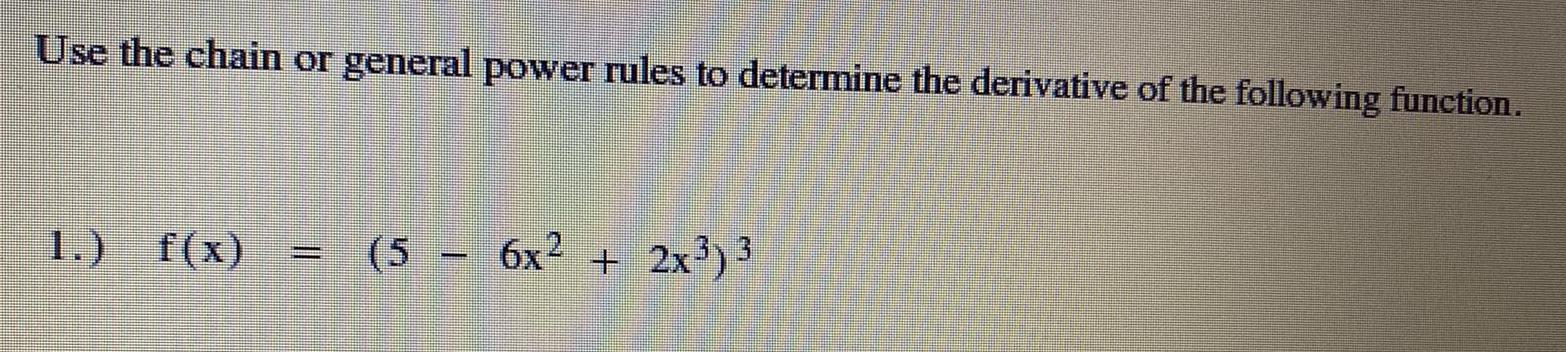Calculus
Differentiation
Use the chain or general power rules to determine the derivative of the following function. f(x)=(5-6x²+2x³)³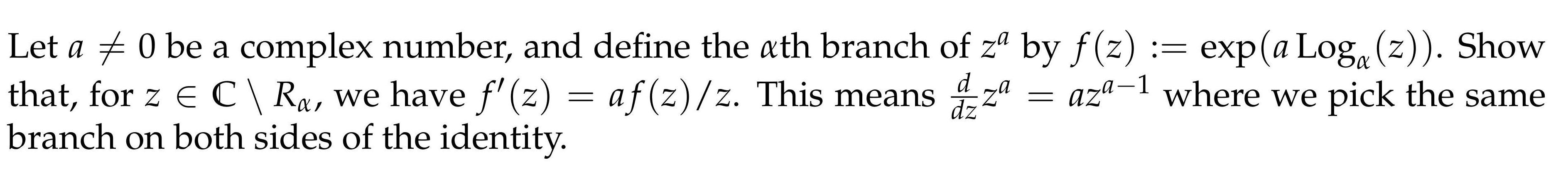Calculus
Differentiation
Let a≠0 be a complex number, and define the αth branch of zª by f(z) := exp(aLogα(z)). Show that, for z € C \ Rα, we have ƒ'(z) = af(z)/z. This means d/dzzª= aza-1 where we pick the same branch on both sides of the identity.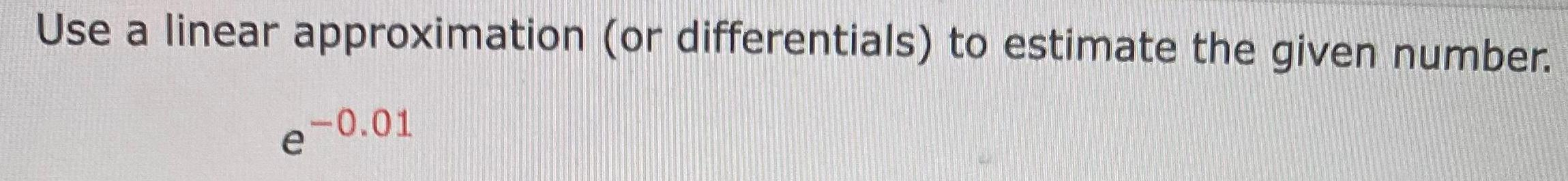Calculus
Differentiation
Use a linear approximation (or differentials) to estimate the given number. e^-0.01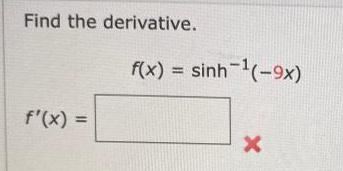Calculus
Differentiation
Find the derivative. f(x) = sinh-¹(-9x) f'(x) =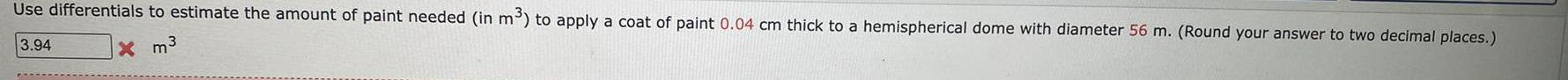Calculus
Differentiation
Use differentials to estimate the amount of paint needed (in m³) to apply a coat of paint 0.04 cm thick to a hemispherical dome with diameter 56 m. (Round your answer to two decimal places.)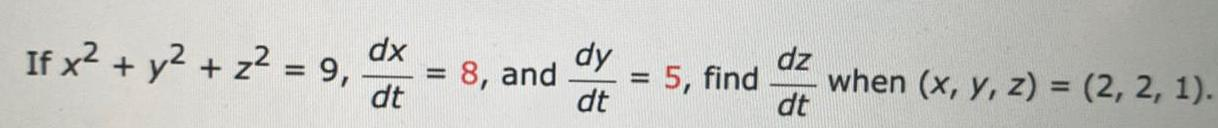Calculus
Differentiation
If x² + y² + z² = 9, dx/dt= 8, and dy/dt =5, find dz/dt when (x, y, z) = (2, 2, 1).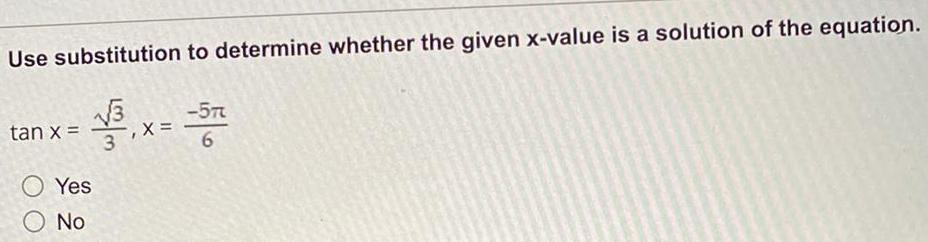Calculus
Differentiation
Use substitution to determine whether the given x-value is a solution of the equation. tan x =√3/3, x= -5π/6 Yes No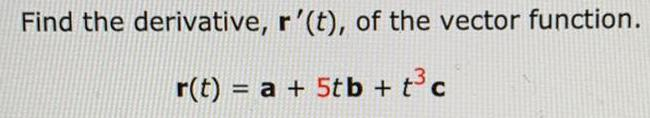Calculus
Differentiation
Find the derivative, r'(t), of the vector function. r(t) = a + 5tb + t³c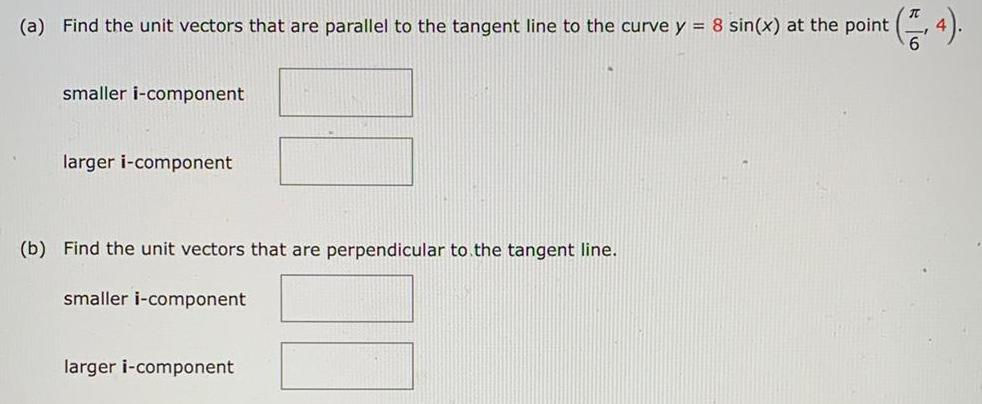Calculus
Differentiation
(a) Find the unit vectors that are parallel to the tangent line to the curve y = 8 sin(x) at the point (π/6 ,4). smaller i-component larger i-component (b) Find the unit vectors that are perpendicular to the tangent line. smaller i-component larger i-component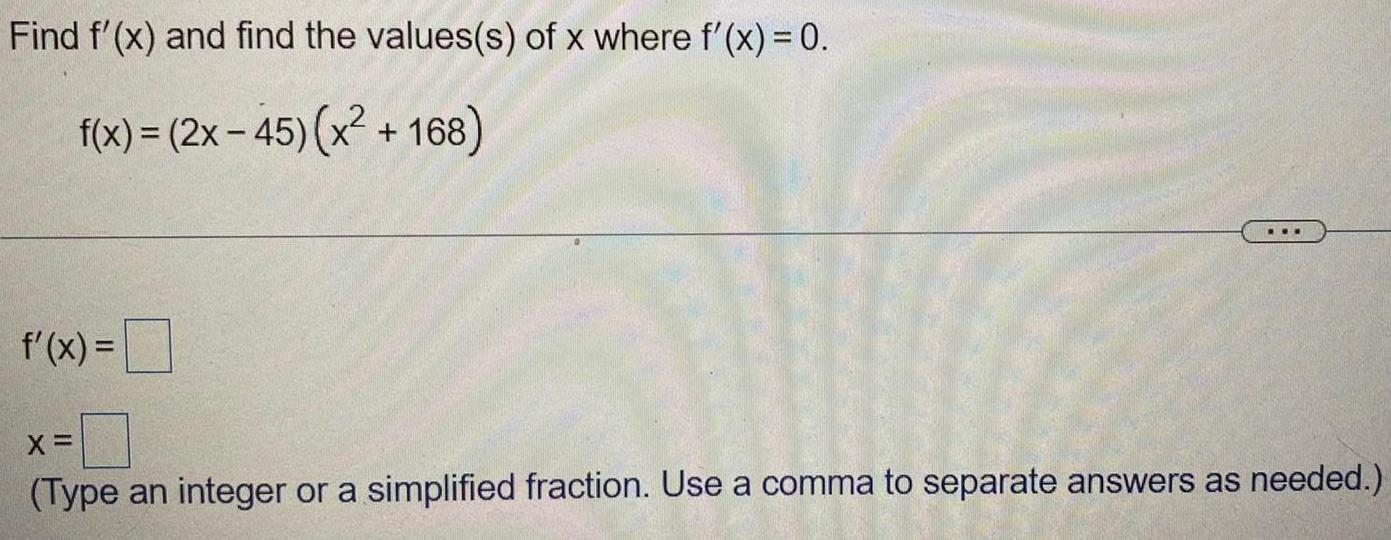Calculus
Differentiation
Find f'(x) and find the values(s) of x where f'(x) = 0. f(x) = (2x - 45) (x² +168) f'(x) = x= (Type an integer or a simplified fraction. Use a comma to separate answers as needed.)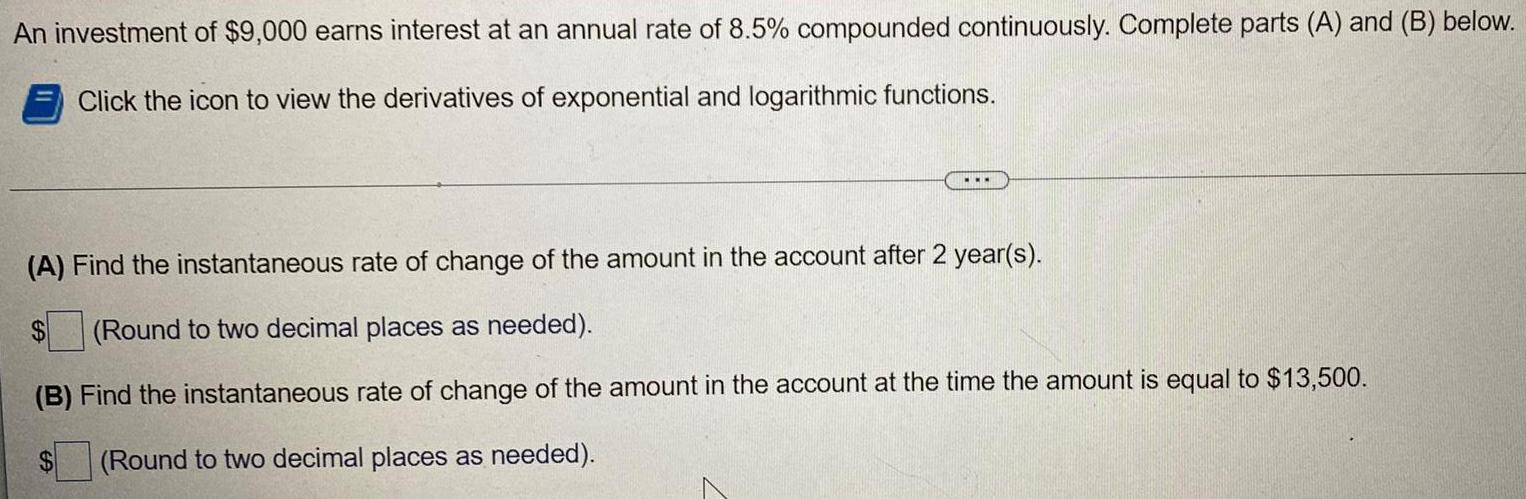Calculus
Differentiation
An investment of \$9,000 earns interest at an annual rate of 8.5% compounded continuously. Complete parts (A) and (B) below. (A) Find the instantaneous rate of change of the amount in the account after 2 year(s). \$ (Round to two decimal places as needed). (B) Find the instantaneous rate of change of the amount in the account at the time the amount is equal to \$13,500. (Round to two decimal places as needed). \$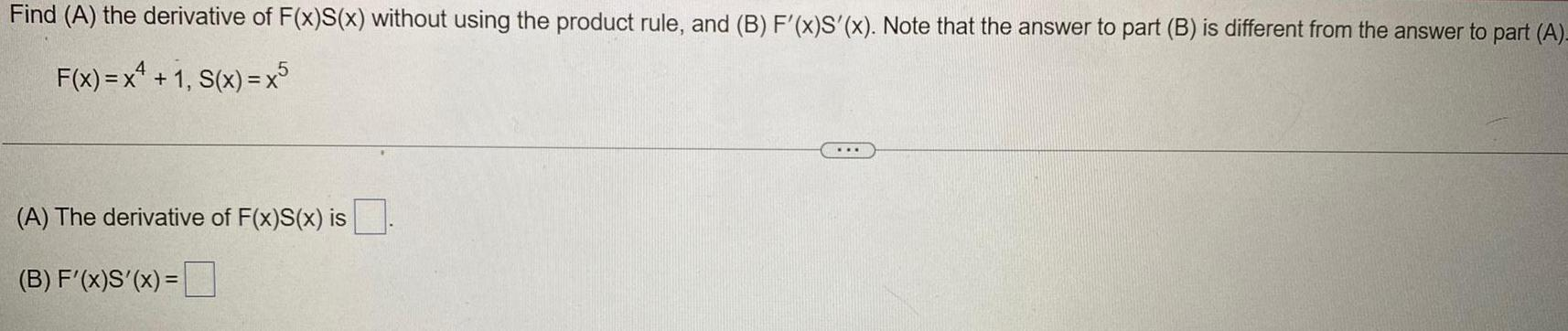Calculus
Differentiation
Find (A) the derivative of F(x)S(x) without using the product rule, and (B) F'(x)S'(x). Note that the answer to part (B) is different from the answer to part (A)- F(x) = x^4 + 1, S(x) = x^5 (A) The derivative of F(x)S(x) is. (B) F'(x)S'(x) =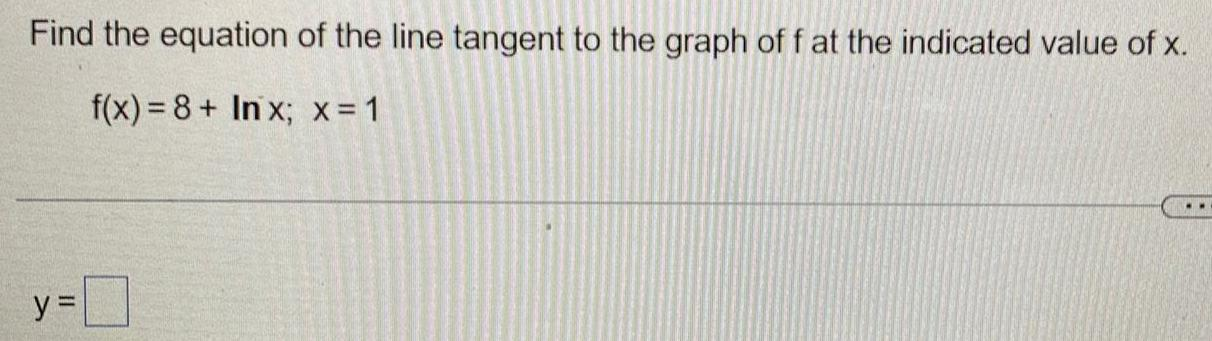Calculus
Differentiation
Find the equation of the line tangent to the graph of f at the indicated value of x. f(x)= 8+ Inx; x = 1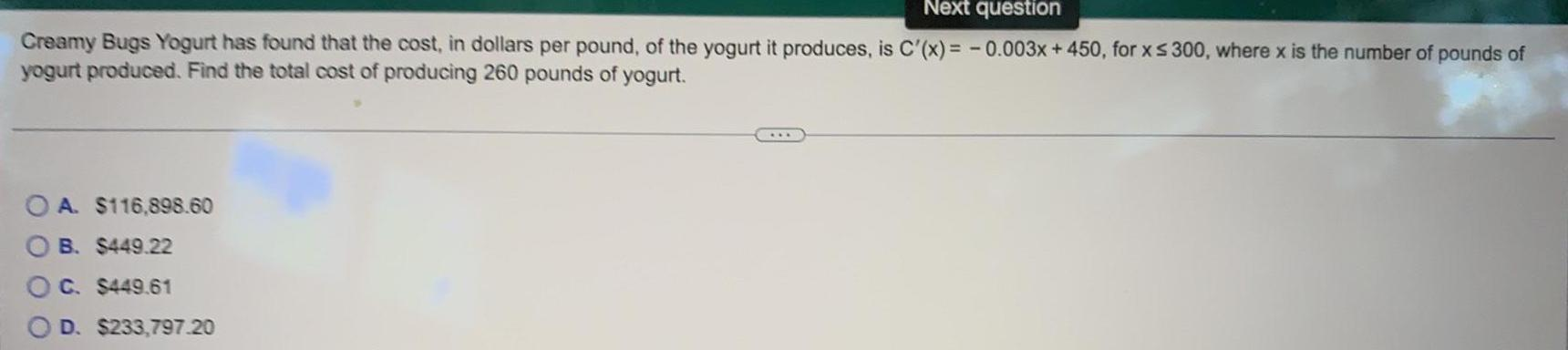Calculus
Differentiation
Creamy Bugs Yogurt has found that the cost, in dollars per pound, of the yogurt it produces, is C'(x) = -0.003x + 450, for x ≤ 300, where x is the number of pounds of yogurt produced. Find the total cost of producing 260 pounds of yogurt. A. \$116,898.60 B. \$449.22 C. \$449.61 D. \$233,797.20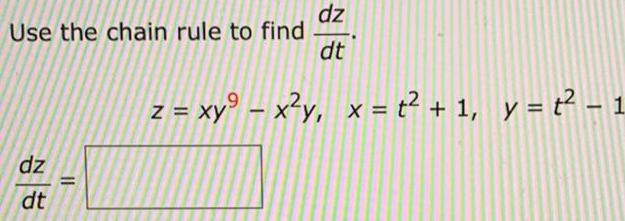Calculus
Differentiation
Use the chain rule to find dz/dt z = xy^9 - x^2y, x = t^2 + 1, y = t^2 - 1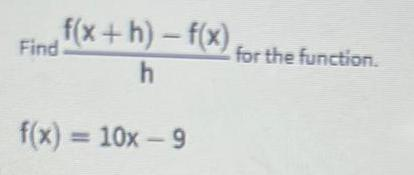Calculus
Differentiation
Find f(x+h) - f(x) / h for the function f(x) = 10x - 9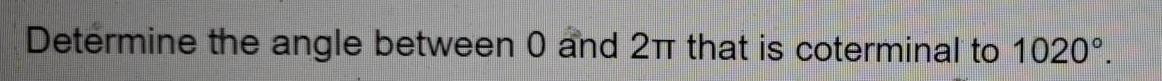Calculus
Differentiation
Determine the angle between 0 and 2π that is coterminal to 1020°.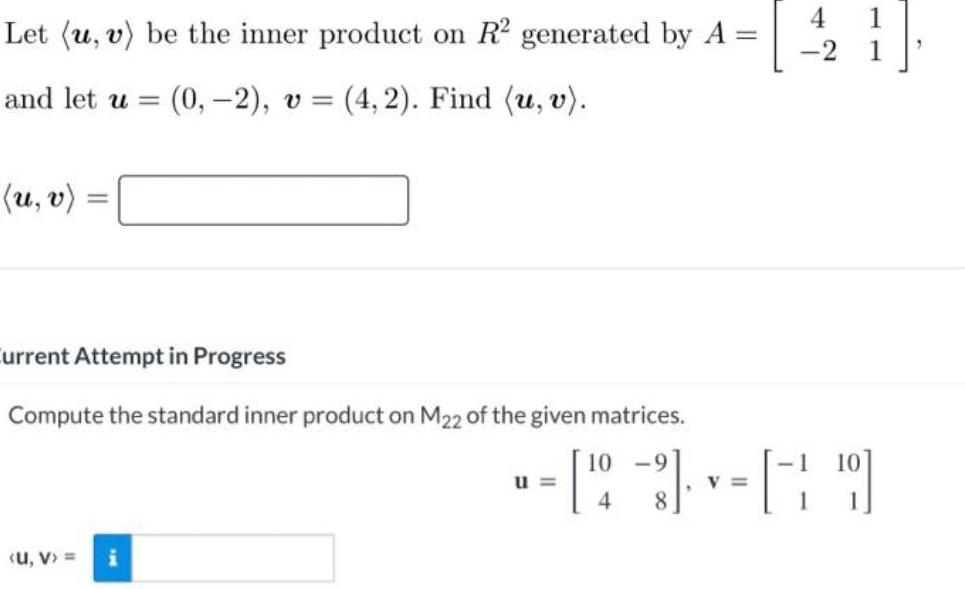Calculus
Differentiation
Let (u, v) be the inner product on R² generated by A = [4 1] [-2 1] and let u = (0, -2), v = (4,2). Find (u, v). (u, v) = Current Attempt in Progress Compute the standard inner product on M22 of the given matrices.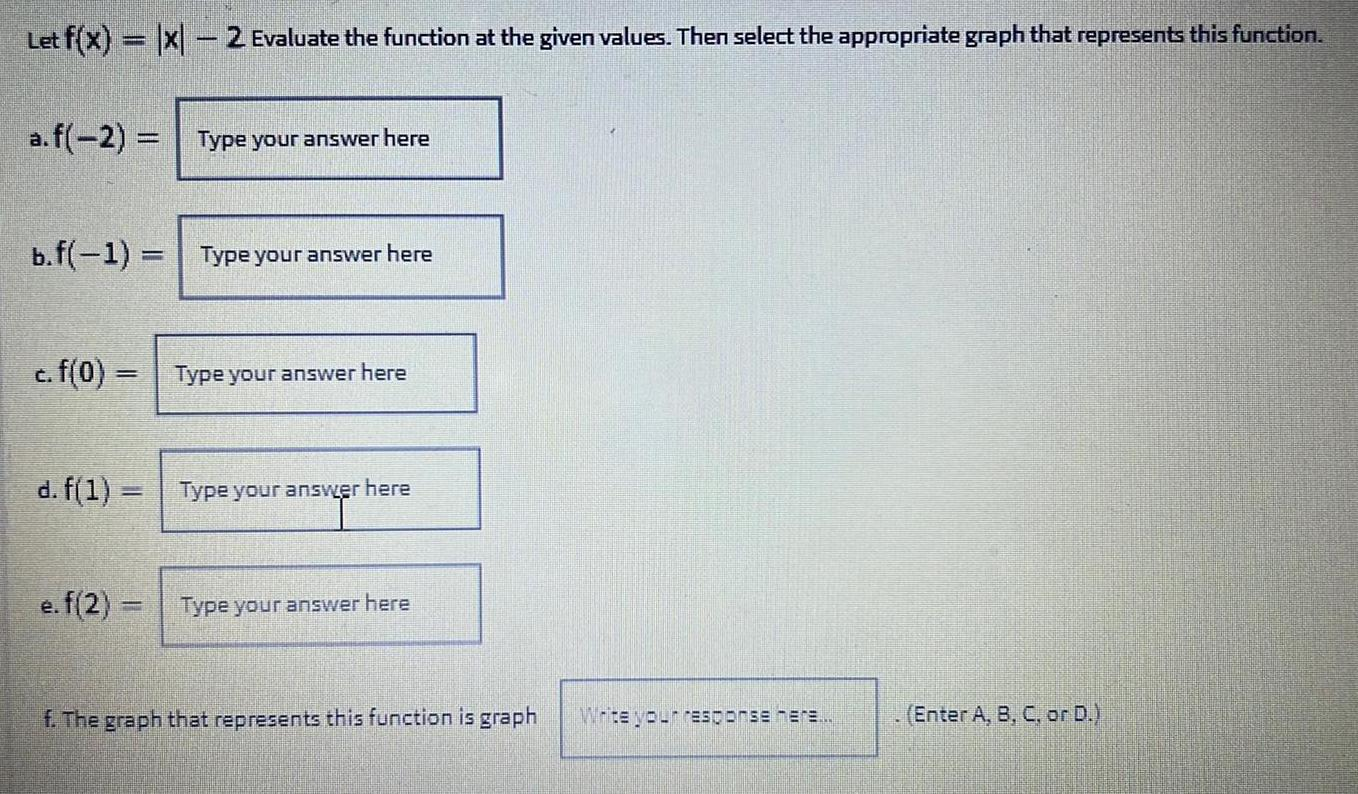Calculus
Differentiation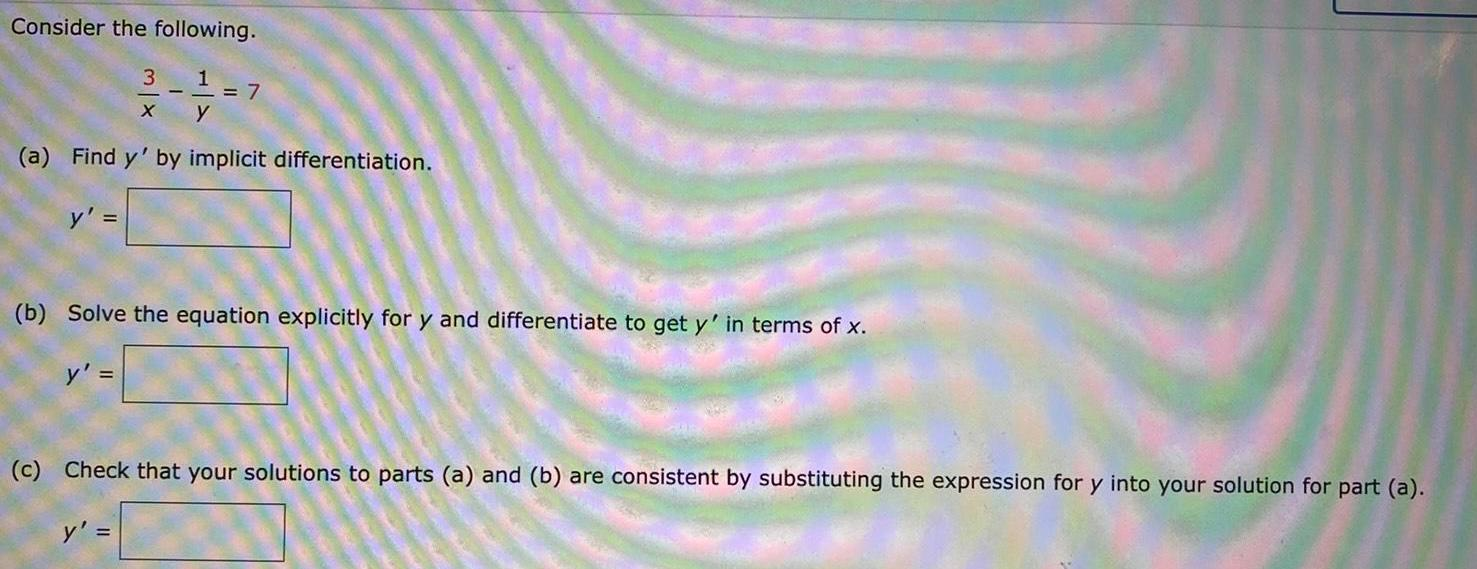Calculus
Differentiation
Consider the following. 3/x - 1/y =7 (a) Find y' by implicit differentiation. y' = (b) Solve the equation explicitly for y and differentiate to get y' in terms of x. y' = (c) Check that your solutions to parts (a) and (b) are consistent by substituting the expression for y into your solution for part (a). y' =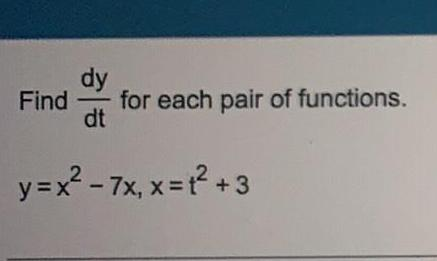Calculus
Differentiation
Find dy/dt for each pair of functions. y=x²-7x, x = t² +3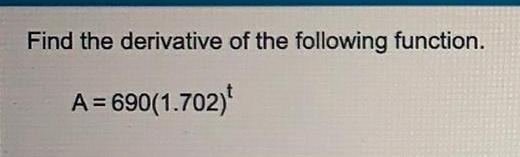Calculus
Differentiation
Find the derivative of the following function. A = 690(1.702)^t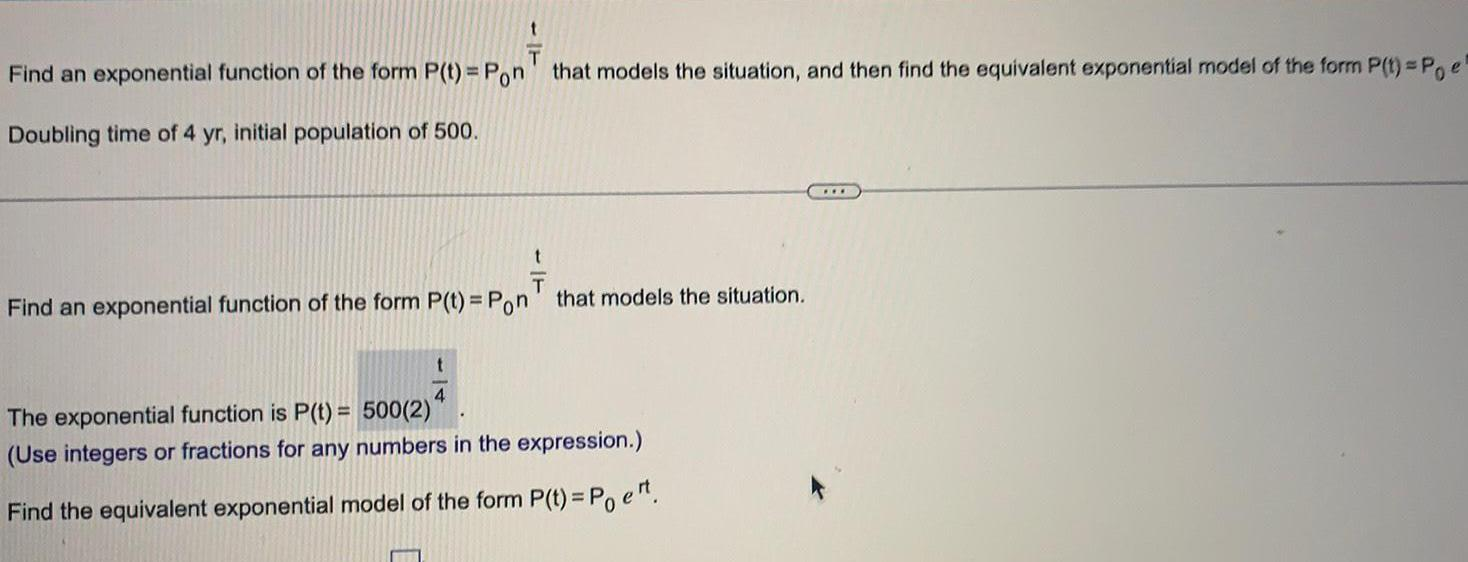Calculus
Differentiation
Find an exponential function of the form P(t)= Pon^1/T that models the situation, and then find the equivalent exponential model of the form P(t)=Poe. Doubling time of 4 yr, initial population of 500. Find an exponential function of the form P(t) = Pon^1/T that models the situation. The exponential function is P(t) = 500(2)^1/4 (Use integers or fractions for any numbers in the expression.) Find the equivalent exponential model of the form P(t) = Poe^rt.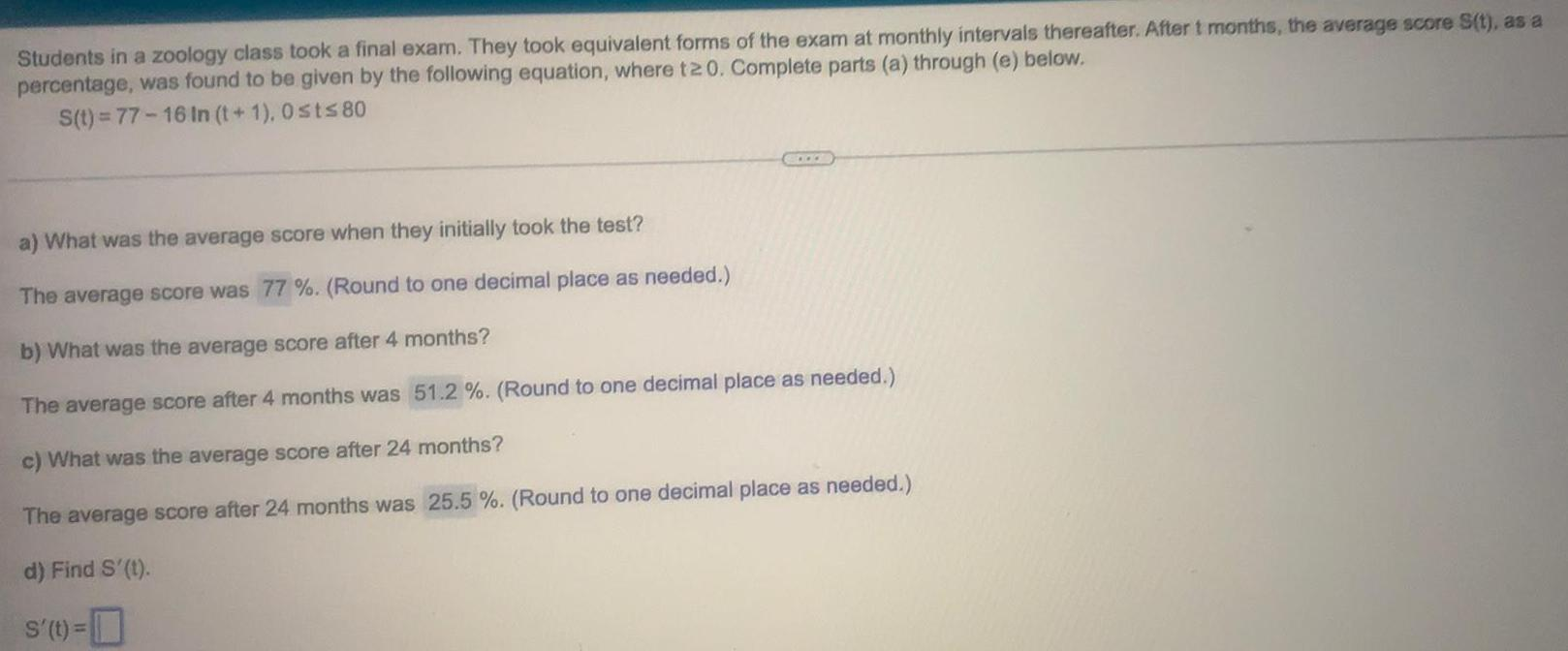Calculus
Differentiation
Students in a zoology class took a final exam. They took equivalent forms of the exam at monthly intervals thereafter. After t months, the average score S(t), as a percentage, was found to be given by the following equation, where t20. Complete parts (a) through (e) below. S(t)=77-16 In (t+1), 0≤t≤80 a) What was the average score when they initially took the test? The average score was 77%. (Round to one decimal place as needed.) b) What was the average score after 4 months? The average score after 4 months was 51.2 %. (Round to one decimal place as needed.) c) What was the average score after 24 months? The average score after 24 months was 25.5 %. (Round to one decimal place as needed.) d) Find S'(t). S'(t)=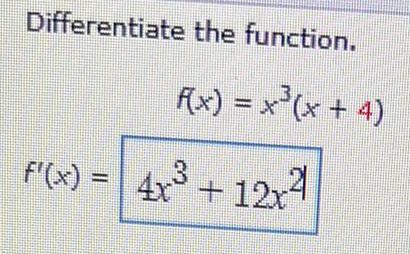Calculus
Differentiation
Differentiate the function. f(x) = x²(x + 4) f'(x) = 4x³ + 12x²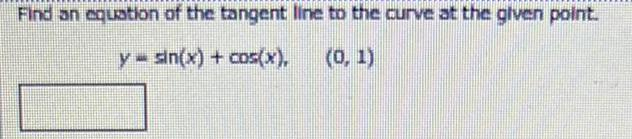Calculus
Differentiation
Find an equation of the tangent line to the curve at the given point. y = sin(x) + cos(x), (0, 1)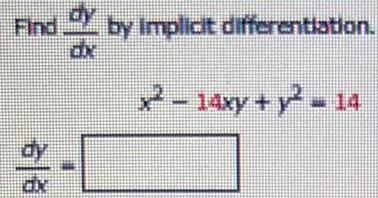Calculus
Differentiation
Find dy/dx by implicit differentiation. x² - 14xy + y² = 14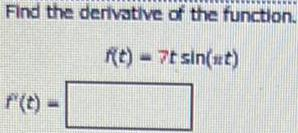Calculus
Differentiation
Find the derivative of the function. f(t)- 7t sin(ut)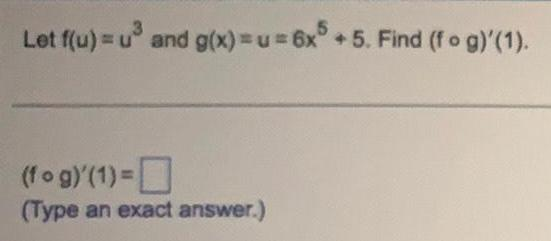Calculus
Differentiation
Let f(u) = u³ and g(x)=u=6x^5 +5. Find (fog)'(1). (fog)'(1) = (Type an exact answer.)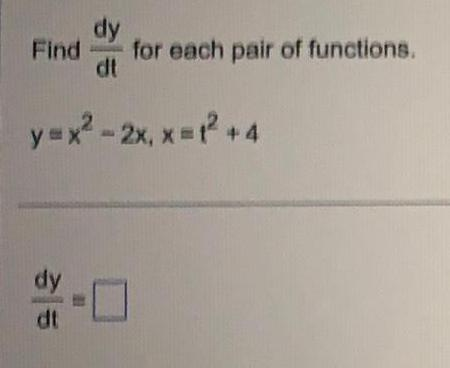Calculus
Differentiation
Find dy/dt for each pair of functions. y=x²-2x, x= t² +4 dy/dt=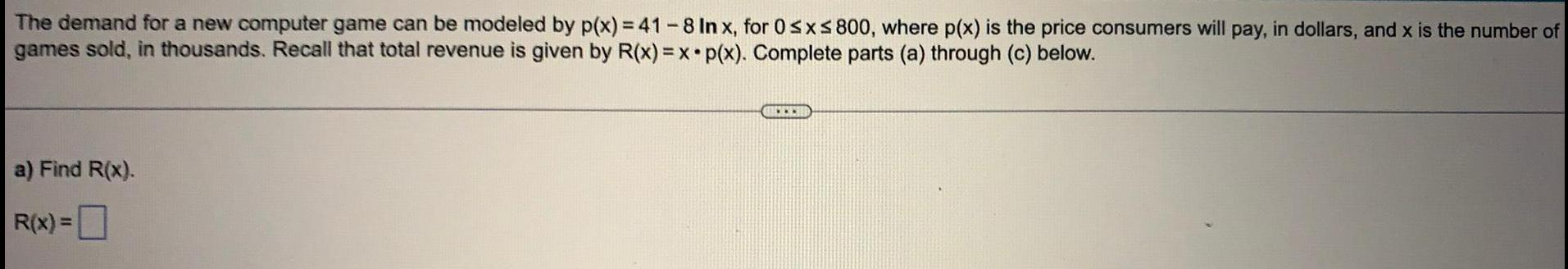Calculus
Differentiation
The demand for a new computer game can be modeled by p(x) = 41-8 In x, for 0 ≤x≤ 800, where p(x) is the price consumers will pay, in dollars, and x is the number of games sold, in thousands. Recall that total revenue is given by R(x)=x p(x). Complete parts (a) through (c) below.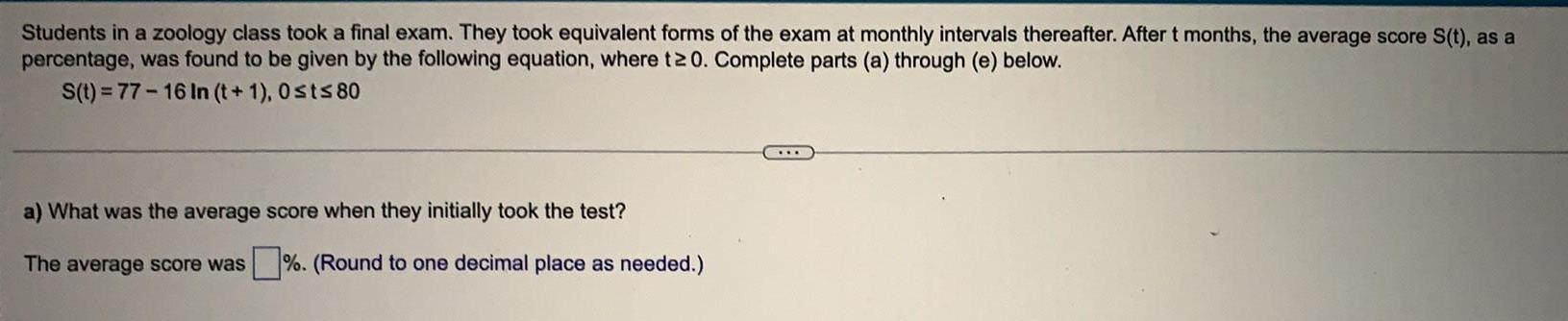Calculus
Differentiation
Students in a zoology class took a final exam. They took equivalent forms of the exam at monthly intervals thereafter. After t months, the average score S(t), as a percentage, was found to be given by the following equation, where t20. Complete parts (a) through (e) below. S(t)=77-16 In (t+1), 0 0 ≤ t≤ 80 a) What was the average score when they initially took the test? The average score was%. (Round to one decimal place as needed.)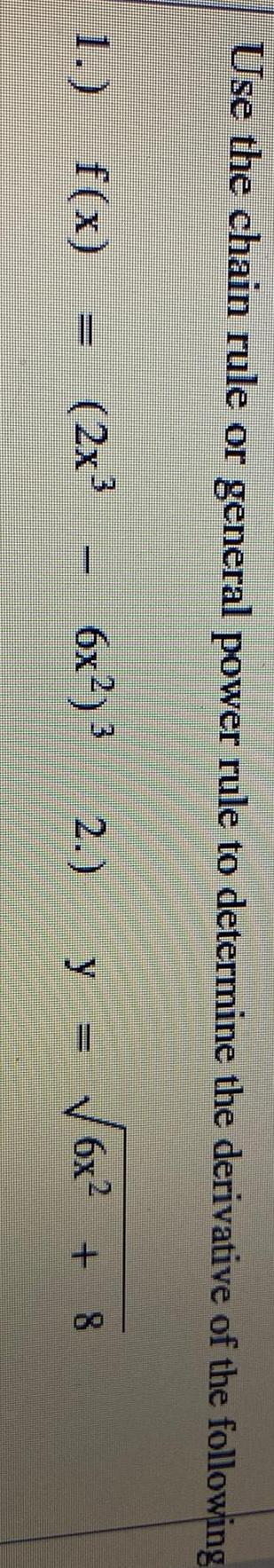Calculus
Differentiation
Use the chain rule or general power rule to determine the derivative of the following f(x)=(2x³-6x²)^ 3 2.) y=√6x² +8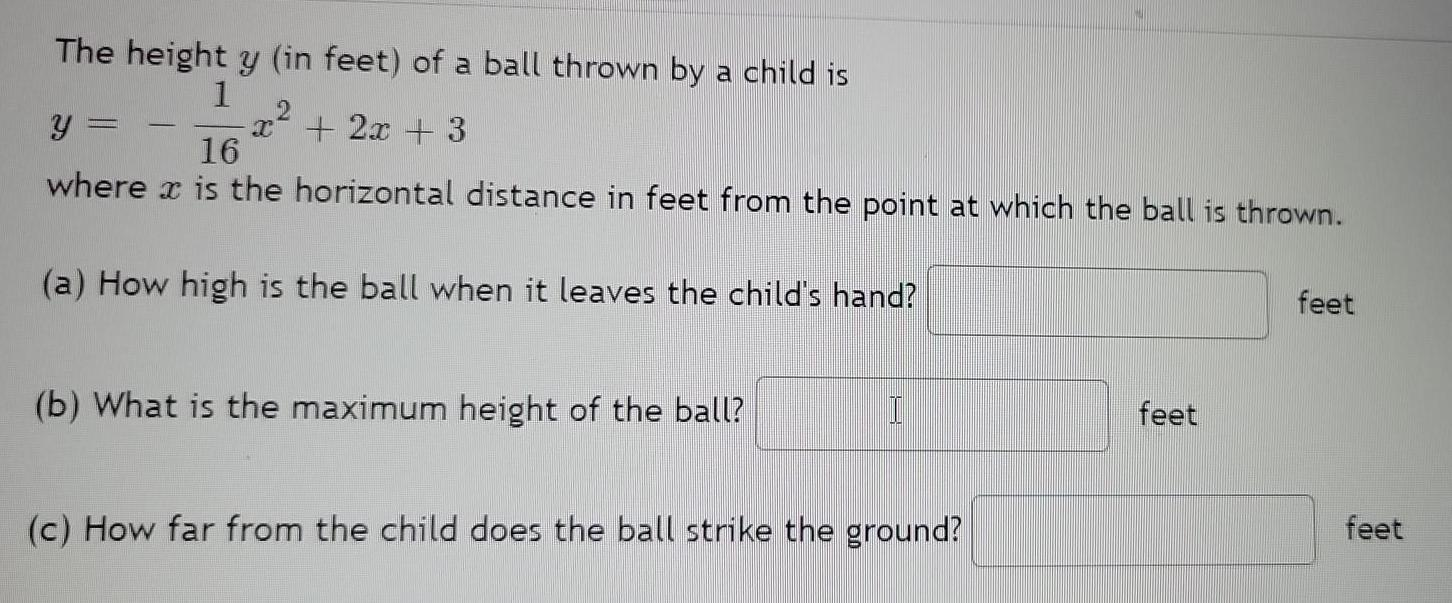Calculus
Differentiation
The height y (in feet) of a ball thrown by a child is y = -1/16 x2+2x+3 where is the horizontal distance in feet from the point at which the ball is thrown. (a) How high is the ball when it leaves the child's hand? (b) What is the maximum height of the ball? (c) How far from the child does the ball strike the ground?